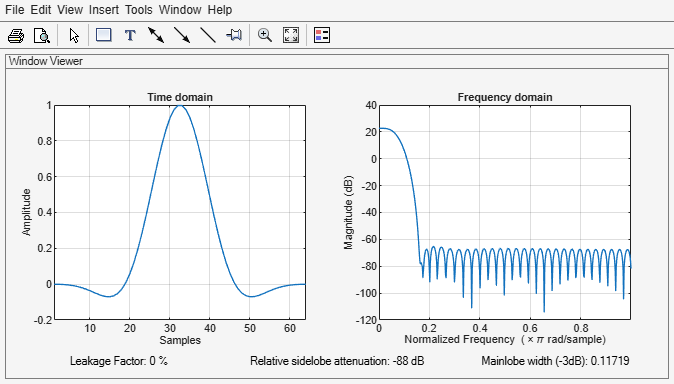# flattopwin

Flat top weighted window

## Syntax

w = flattopwin(L)
w = flattopwin(L,sflag)

## Description

example

w = flattopwin(L) returns an L-point symmetric flat top window 
w = flattopwin(L,sflag) returns an L-point symmetric flat top window using the window sampling method specified by sflag.

## Examples

collapse all

Create a 64-point symmetric flat top window. View the result using wvtool.

N = 64; w = flattopwin(N); wvtool(w)## Input Arguments

collapse all

Window length, specified as a positive integer.

Data Types: single | double

Window sampling method, specified as:

• 'symmetric' — Use this option when using windows for filter design.

• 'periodic' — This option is useful for spectral analysis because it enables a windowed signal to have the perfect periodic extension implicit in the discrete Fourier transform. When 'periodic' is specified, the function computes a window of length L + 1 and returns the first L points.

## Output Arguments

collapse all

Flat top window, returned as a column vector.

## Algorithms

Flat top windows are summations of cosines. The coefficients of a flat top window are computed from the following equation:

$w\left(n\right)={a}_{0}-{a}_{1}\mathrm{cos}\left(\frac{2\pi n}{L-1}\right)+{a}_{2}\mathrm{cos}\left(\frac{4\pi n}{L-1}\right)-{a}_{3}\mathrm{cos}\left(\frac{6\pi n}{L-1}\right)+{a}_{4}\mathrm{cos}\left(\frac{8\pi n}{L-1}\right),$

where $0\le n\le L-1$. The coefficient values are:

CoefficientValue
a00.21557895
a10.41663158
a20.277263158
a30.083578947
a40.006947368

Flat top windows have very low passband ripple (< 0.01 dB) and are used primarily for calibration purposes. Their bandwidth is approximately 2.5 times wider than a Hann window.

 D’Antona, Gabriele, and A. Ferrero. Digital Signal Processing for Measurement Systems. New York: Springer Media, 2006, pp. 70–72.

 Gade, Svend, and Henrik Herlufsen. “Use of Weighting Functions in DFT/FFT Analysis (Part I).” Windows to FFT Analysis (Part I): Brüel & Kjær Technical Review. Vol. x, Number 3, 1987, pp. 1–28.